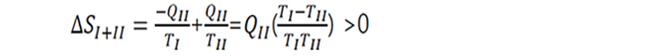## Want to keep learning?

This content is taken from the Hanyang University's online course, Thermodynamics in Energy Engineering. Join the course to learn more.
3.6

# Entropy is not conserved

Entropy
• Unlike energy functions, entropy is not conserved in natural process or in isolated systems.
• For example, consider heat transfer between two objects with different temperature TI and TII (TI > TI)in an isolated system. The two objects are connected by a thermal conductor such as a copper wire. The heat will flow from the high temperature side (I) to the low temperature side (II). Assuming no heat loss, the heat from I will go into II, thus $$Q$$$$I$$=−$$Q$$$$II$$• The internal energy change of the whole system (I+II) is thus zero.
∆$$U$$$$I+II$$=$$∆U$$$$I$$+$$∆U$$$$II$$=$$Q$$$$I$$+$$Q$$$$II$$=0
The energy is conserved.
• Let’s consider the entropy change of the whole system (I+II).Insert the relation $$Q$$$$I$$=−$$Q$$$$II$$The entropy of this isolated system is not conserved. This heat flow is spontaneous and irreversible and the irreversibility cause entropy increase.